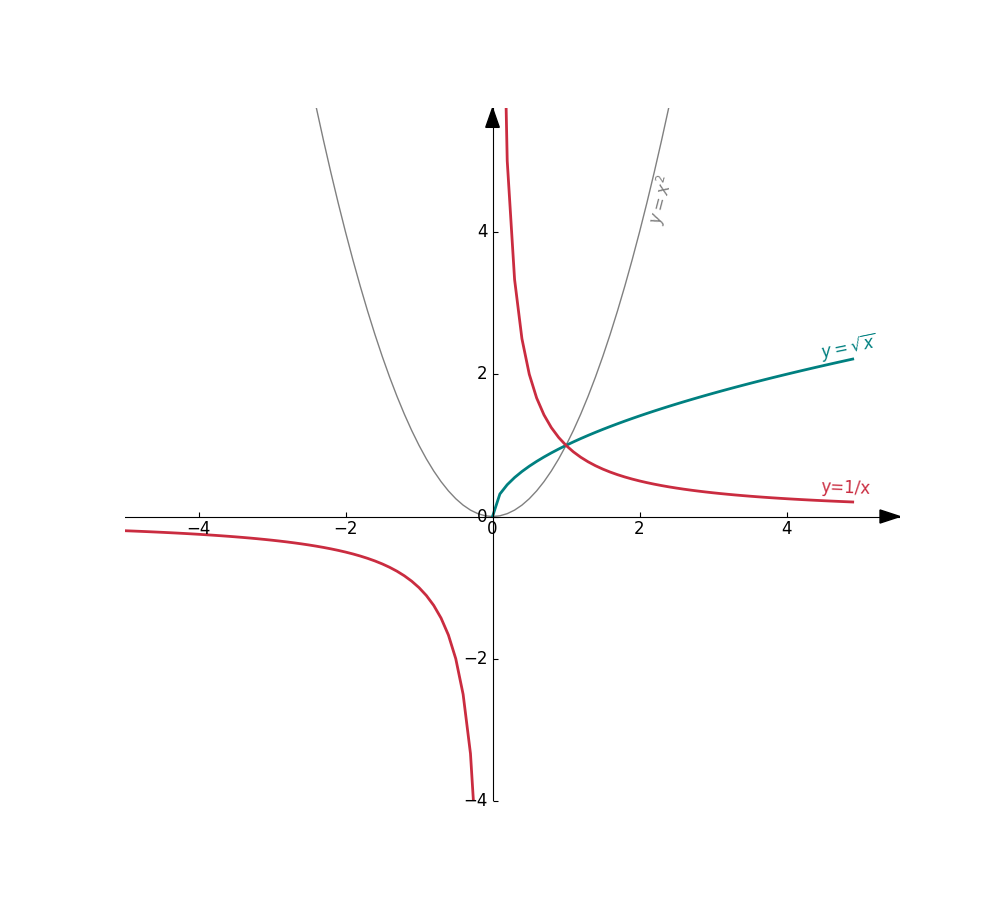# Python: Drawing Function Curves in a Cartesian Coordinate System

New PyGeom Tutorial! How to draw curves from functions. Because we must be at least tens of people out there who want to do visual 2D mathematics in Python…right?

### Step 3: Create your functions and draw them

It is pretty straightforward from there. Let’s create three functions for testing:

$$f(x) = x^2; \quad g(x) = \sqrt{x}; \quad h(x) = \frac{1}{x}$$

The entire code + results is shown below

import math
import numpy as np

from pygeom import Axes, Point, Curve

def f(x):
y = math.pow(x, 2)
return y

def g(x):
if x < 0:
y = np.nan
else:
y = math.sqrt(x)
return y

def h(x):
y = 1/x
return y

# Create the cartesian axis
axes = Axes(xlim=(-5,5), ylim=(-4,5.2), figsize=(10,9))

# Curve of y = x^2
c1 = Curve(f, label=r'$y=x^2$', color='grey')
c2 = Curve(g, label=r'$y=\sqrt{x}$', linewidth=2, color='#008080')